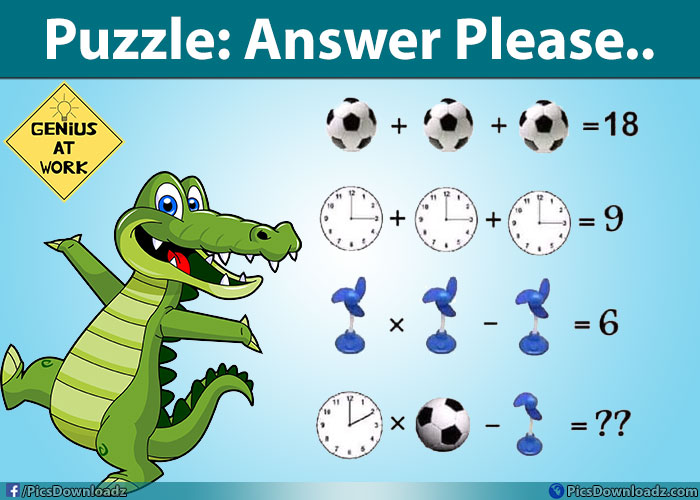This post may contain affiliate links. This means if you click on the link and purchase the item, We will receive an affiliate commission at no extra cost to you. See Our Affiliate Policy for more info.

Solve this Football, Watch, & Fan image Math Puzzle – Fun Brain teasers Puzzles

0
2440

Solve this Picture Puzzles Image – Genius Math and Brainteasers Puzzles

Hello, Friends! Solve this image puzzle if you are a genius. In this puzzle there are three images given; football, watch and fan. You will have to find out of each value and then solve the given equation at the end of the image.

Math & Brainteasers Image PizzlesGot it? let see what you get.

Well, there is a trick behind this puzzle too. If you check the image carefully, then you will find some changes in the last equation of this puzzle.

>>First Step:

In this step, consider Football = B

Therefore, B + B + B = 18,   3B = 18,  B = 6 (with six black spots)

If you noticed, there are six black spots in the football in first equation but in last equation, there are only three black spots. Which mean, for last equation Football value will be, B = 3

>> Second Step:

Second equation has three watches, but check their times 3 O’clock, So 3 + 3 + 3 =9.

But in last equation, the time is 2 O’clock. So watch value in W= 2 for the last step

>>Third Step:

Three Fans, each fan have three wings. But in the last step, there are only 2 wings, So F = 2

>>Fourth Step:

Therefore; put all values in this equation W x B – F =?,

∴ 2 × 3 – 2 = 4

SHARE & LIKE

Hope you find this puzzle interesting. For more fun, connect with us on facebook and get interesting updates on your timeline. Take care, Enjoy!

Check out some other puzzles from our website:

95% will fail to answer this Counter Strike Puzzle – Math Puzzles Only for Geniuses

95% Answer this Triangle Puzzle wrong! Brain Teasers Puzzles

How many numbers are between 100 to 200? – Math Puzzles Images

Search items:

Solve the Puzzles, Find Puzzles Answer, Math Game, Brain Teasers Puzzles, Logic Puzzles, Riddles, Math Puzzles, Image Riddles, Riddle Game, Whatsapp Puzzles, Puzzles for Share, Puzzles for Facebook, Whatsapp Riddles, Brain Game Riddles, Puzzles for Whatsapp, Brain Training Puzzles, Challenge your Brain, Confusing Riddles, Difficult Brain Teasers, Difficult Math Puzzles, Fun Brain Puzzles, Fun Riddles Brain Teasers, Mind Puzzles, Math Riddle Answers, Math Fun Questions, Mind Puzzles Riddles, Puzzles and Mind Games, Riddle Brain Teasers, Riddle Websites, Riddles and Mind Games, Riddles and Puzzles, Solve this if you are a genius, Genius Riddles, Only for Genius, Only for Genius Puzzles, Math Puzzle with Answer, Genius at Work Puzzles, Interesting Puzzle Games,